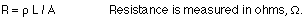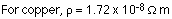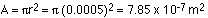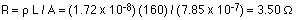## Batteries, current, and Ohm's law

7-10-00

Section 18.1 - 18.4

### Batteries and EMF

Capacitors are very good at storing charge for short time periods, and they can be charged and recharged very quickly. There are many applications, however, where it's more convenient to have a slow-but-steady flow of charge; for these applications batteries are used.

A battery is another device for storing charge (or, put another way, for storing electrical energy). A battery consists of two electrodes, the anode (negative) and cathode (positive. Usually these are two dissimilar metals such as copper and zinc. These are immersed in a solution (sometimes an acid solution). A chemical reaction results in a potential difference between the two terminals.

When the battery is connected to a circuit, electrons produced by the chemical reaction at the anode flow through the circuit to the cathode. At the cathode, the electrons are consumed in another chemical reaction. The circuit is completed by positive ions (H+, in many cases) flowing through the solution in the battery from the anode to the cathode.

The voltage of a battery is also known as the emf, the electromotive force. This emf can be thought of as the pressure that causes charges to flow through a circuit the battery is part of. This flow of charge is very similar to the flow of other things, such as heat or water.

A flow of charge is known as a current. Batteries put out direct current, as opposed to alternating current, which is what comes out of a wall socket. With direct current, the charge flows only in one direction. With alternating current, the charges slosh back and forth, continually reversing direction.

The Duracell web site has a nice explanation of how batteries work.

### Current and Drift velocity

An electric current, which is a flow of charge, occurs when there is a potential difference. For a current to flow also requires a complete circuit, which means the flowing charge has to be able to get back to where it starts. Current (I) is measured in amperes (A), and is the amount of charge flowing per second.

current : I = q / t, with units of A = C / s

When current flows through wires in a circuit, the moving charges are electrons. For historical reasons, however, when analyzing circuits the direction of the current is taken to be the direction of the flow of positive charge, opposite to the direction the electrons go. We can blame Benjamin Franklin for this. It amounts to the same thing, because the flow of positive charge in one direction is equivalent to the flow of negative charge in the opposite direction.

When a battery or power supply sets up a difference in potential between two parts of a wire, an electric field is created and the electrons respond to that field. In a current-carrying conductor, however, the electrons do not all flow in the same direction. In fact, even when there is no potential difference (and therefore no field), the electrons are moving around randomly. This random motion continues when there is a field, but the field superimposes onto this random motion a small net velocity, the drift velocity. Because electrons are negative charges, the direction of the drift velocity is opposite to the electric field.

In a typical case, the drift velocity of electrons is about 1 mm / s. The electric field,on the other hand, propagates much faster than this, more like 108 m / s.

### Electrical resistance

Voltage can be thought of as the pressure pushing charges along a conductor, while the electrical resistance of a conductor is a measure of how difficult it is to push the charges along. Using the flow analogy, electrical resistance is similar to friction. For water flowing through a pipe, a long narrow pipe provides more resistance to the flow than does a short fat pipe. The same applies for flowing currents: long thin wires provide more resistance than do short thick wires.

The resistance (R) of a material depends on its length, cross-sectional area, and the resistivity (r ), a number that depends on the material:The resistivity and conductivity are inversely related. Good conductors have low resistivity, while poor conductors (insulators) have resistivities that can be 20 orders of magnitude larger.

Resistance also depends on temperature, usually increasing as the temperature increases. For reasonably small changes in temperature, the change in resistivity, and therefore the change in resistance, is proportional to the temperature change. This is reflected in the equations:At low temperatures some materials, known as superconductors, have no resistance at all. Resistance in wires produces a loss of energy (usually in the form of heat), so materials with no resistance produce no energy loss when currents pass through them.

### Ohm's Law

In many materials, the voltage and resistance are connected by Ohm's Law:

Ohm's Law : V = IR

The connection between voltage and resistance can be more complicated in some materials.These materials are called non-ohmic. We'll focus mainly on ohmic materials for now, those obeying Ohm's Law.

### Example

A copper wire has a length of 160 m and a diameter of 1.00 mm. If the wire is connected to a 1.5-volt battery, how much current flows through the wire?

The current can be found from Ohm's Law, V = IR. The V is the battery voltage, so if R can be determined then the current can be calculated. The first step, then, is to find the resistance of the wire:L is the length, 1.60 m. The resistivity can be found from the table on page 535 in the textbook.The area is the cross-sectional area of the wire. This can be calculated using:The resistance of the wire is then:The current can now be found from Ohm's Law:

I = V / R = 1.5 / 3.5 = 0.428 A